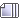###Author Topic: Grumpy's Algebraic therory  (Read 5885 times)

1/0

I win.

#### fireball

x=y
x^2=y^2
xy=y^2
xy-x^2=y^2-x^2
-x(x-y)=(x+y)(x-y)
-x=x+y
2x=y
2x=x
2=1
1=0

No me

#### Deoxy99

1/2 of m = n
1/2 of w = v
1/2 of o + l = {b,d}

I win.

1/2 of (o x I) = {W,V} would make more sense!

You cannot use another two letters for the equals if you want it to be hard!

I WIN!

2 + 2 = 22

#### WRB852

x=y
x^2=y^2
xy=y^2
xy-x^2=y^2-x^2
-x(x-y)=(x+y)(x-y)
-x=x+y
2x=y
2x=x
2=1
1=0

No me
you can't divide by zero

#### LaCuckooRacha

x=y
x^2=y^2
xy=y^2
xy-x^2=y^2-x^2
-x(x-y)=(x+y)(x-y)
-x=x+y
2x=y
2x=x
2=1
1=0

No me

Lets go through that with numbers
x = 2 y = 2
2 = 2
2^2 = 2^2
2 x 2 = 2^2
2 x 2 - 2^2 = 2^2 - 2^2
-2 x (2 - 2) = (2 + 2) x (2 - 2)
-2 = (2 + 2)
-2 = 4

Really, all you need is;
-2 x (2 - 2) = (2 + 2) x (2 - 2)
because you can cancel the (2 - 2) from each side,
-2 = (2 + 2)
Meaning -2 = 4, -3 = 6, -4 = 8
O_o  Math class is meaningless.

#### Deep2

The reason you can't divide by 0 is because zero is not a number. 0 is nothing at all, so how does it make sense to divide something by a non number? It's impossible.

#### Inv3rted

The reason you can't divide by 0 is because zero is not a number. 0 is nothing at all, so how does it make sense to divide something by a non number? It's impossible.

Zero is a number. It's an integer.

#### Deep2

Zero is a number. It's an integer.

Then how come you can't have a positive OR negative zero?

-1*(b)=d

#### Deep2

Whoah

0/0^0=1

Did I just solve it?

#### Rughugger

You people forgot one!

8=D             ;D#### Robo Noob

Then how come you can't have a positive OR negative zero?
It's neutral.

#### Rughugger

It's neutral.
It's an absence of value. Much like black really isn't a color but the total absence of all color. Technically the only reason 0 exists is for binary computer programing.